# 前提

### 递归

大部分的递归定义都由三个部分构成：基本情况的定义，递归法则和递归结束的情况，如果定义的对象是无限的，那么可以省略第三个部分。比如说，可以用递归定义的方式来定义如下的一个自然数集上的函数


f(0)=1
n>0,f(n)=n f(n-1).

f(1) = 1
n>1,f(n) = f(n-1)+1;

//n>=1
if(n==1) return 1;
return  f(n-1)+1;


### 斐波那契数列

• F(0) = 0;
• F(1) = 1;
• F(n) = F(n-1)+F(n-2)

if(n == 0) return 0;
if(n==1) return 1;
return  f(n-1)+f(n-2);


if(n==0) return 0;
if(n==1) return 1;

int old = 0;	//f(0)
int last = 1;	//f(1)
int currentValue //当前值
for(int i = 2;i<=n;i++){
currentValue = last+old;
old = last;
last=currentValue;
}


# 算法分类

## 回溯法

比较有趣的是，回溯法没有你们想的那么高大上。有很多资深玩家(耐心玩家)就喜欢用回溯法解决这个问题。每个路口我都存个档，一个路口一个路口的实验，这个路口玩出的结果不让我满意，我就回退到上一个路口，直到玩出我满意的结果为止。


### 迷宫问题

f(i,j)=f(i-1,j)+f(i+1,j)+f(i,j+1)+f(i,j-1),终止条件是strs.length = startPos


package algorithm;

import java.util.Arrays;

/**
* @description: 回溯法
* @author: Ksssss(chenlin @ hoolai.com)
* @time: 2019-12-22 18:01
*/

public class Solution {

//1、第一点，也是重要的一点判断条件是否满足
//2、需要一个数组来记录它的访问记录（路径不能重复进入)
//3、设置一个hasPath，默认为 false
//4、选择一点出发
//5、判断字符串是否完全匹配。如匹配，标记更改为true，并返回
//6、记录字符串匹配开始位置。判断是否满足条件，满足进入步骤7，不满足进入步骤10
//7、访问点记录为true，匹配字符串下标++
//8、进入步骤4并赋值给hasPath
//9、判断hasPath，如果为false 访问点记录为false，匹配字符串下标--
//10、返回hasPath

public boolean hasPath(char[] matrix, int rowLen, int colLen, char[] str) {
if (rowLen < 1 || colLen < 1 || matrix.length == 0 || str.length == 0) {
return false;
}

boolean[] visited = new boolean[rowLen * colLen];
int strPos = 0;
for (int row = 0; row < rowLen; row++) {
for (int col = 0; col < colLen; col++) {
if (hasPathCore(matrix, row, rowLen, col, colLen, str, strPos, visited)) {
return true;
}
}
}
return false;
}

public boolean hasPathCore(char[] matrix, int row, int rowLen, int col, int colLen, char[] str, int strPos, boolean[] visited) {
//指定位置
if (strPos == str.length) {
return true;
}
boolean hasPath = false;
if (row >= 0 && col >= 0 && row < rowLen && col < colLen
&& str[strPos] == matrix[row * colLen + col] && !visited[row * colLen + col]) {
visited[row * colLen + col] = true;
strPos++;

hasPath = hasPathCore(matrix, row + 1, rowLen, col, colLen, str, strPos, visited) ||
hasPathCore(matrix, row - 1, rowLen, col, colLen, str, strPos, visited) ||
hasPathCore(matrix, row, rowLen, col + 1, colLen, str, strPos, visited) ||
hasPathCore(matrix, row, rowLen, col - 1, colLen, str, strPos, visited);

if (!hasPath) {
strPos--;
visited[row * colLen + col] = false;
}
}
return hasPath;
}

public static void main(String[] args) {
Solution solution = new Solution();
System.out.println(solution.hasPath((
"abce" +
"sfcs" +
"adee").toCharArray(), 3, 4, "bcced".toCharArray()));
}
}


## 动态规划

### 01简单背包问题

f(i,cw) = Max(f(i-1,cw),f(i-1,cw+Wi))  （i>0,i<=n,cw+Wi<w）
//i代表当前物品，cw代表当前背包容量,w代表总容量

	int maxCapacity = 0;	//结果放入maxCapacity中
int[] items = {2，2，4，6，3};  //物品重量
int bagCapacity = 9;    //背包容量
public void knapsack01Core(int startPos,int currentCapacity) {
if (currentCapacity == bagCapacity || startPos == items.length) {
if (currentCapacity > maxCapacity) {
maxCapacity = currentCapacity;
}
return;
}

knapsack01Core(startPos + 1,currentCapacity);//选择不装第i个物品
if (startPos >= 0 && startPos < items.length && bagCapacity >= 0 && currentCapacity < bagCapacity) {//选择装第i个物品
if (items[startPos] + currentCapacity <= bagCapacity) {
currentCapacity = items[startPos] + currentCapacity;
knapsack01Core(startPos + 1,currentCapacity);
}
}
}


• 是求最优解吗？
• 可以拆分成若干个小问题吗？
• 小问题之间是否存在重复计算？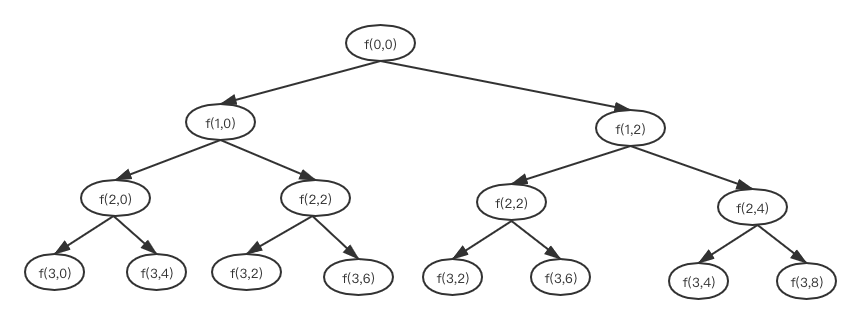### 动态规划版 简单0-1背包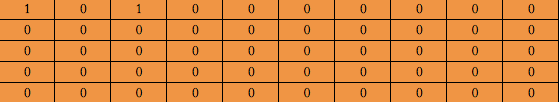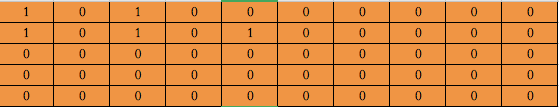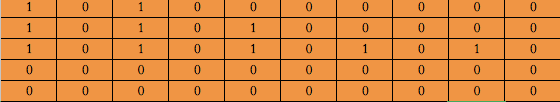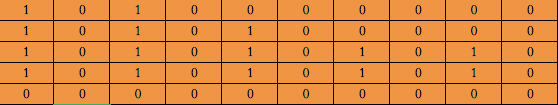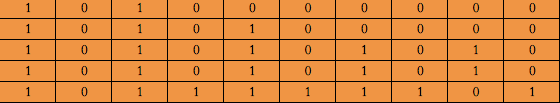//动态规划

//f(i,cw)=Max(f(i-1,cw),f(i-1,cw-Wi))
public int knapsack01Core_3(int[] items, int bagCapacity) {
boolean[][] states = new boolean[items.length][bagCapacity + 1];
states = true;
if (bagCapacity > items) {
states[items] = true;
}
for (int i = 1; i < items.length; i++) {
//不放入背包
for (int j = 0; j <= bagCapacity; j++) {
if (states[i - 1][j]) {
states[i][j] = true;
}
}
//放入背包
for (int j = 0; j <= bagCapacity - items[i]; j++) {
if (states[i - 1][j]) {
states[i][j + items[i]] = true;
}
}
}

for (int i = bagCapacity; i >= 0; i--) {
if (states[items.length - 1][i]) {
return i;
}
}
return 0;
}


public int knapsack01Core_1(int[] items, int bagCapacity) {
boolean[] states = new boolean[bagCapacity + 1];
//特殊处理
states = true;
for (int i = 0; i < items.length; i++) {
//
for (int j = bagCapacity - items[i]; j >= 0; j--) {
if (states[j]) {
states[j + items[i]] = true;
}
}
}

for (int i = bagCapacity; i >= 0; i--) {
if (states[i]) {
return i;
}
}
return 0;
}02-24
08-1127万+
08-2422万+
10-1120万+
07-1528万+
12-2211万+
12-2832万+
11-132万+
05-2918万+
09-234万+
05-081961
07-0637万+
06-1614万+
11-207万+
07-29889
06-059838
©️2020 CSDN 皮肤主题: 大白 设计师:CSDN官方博客